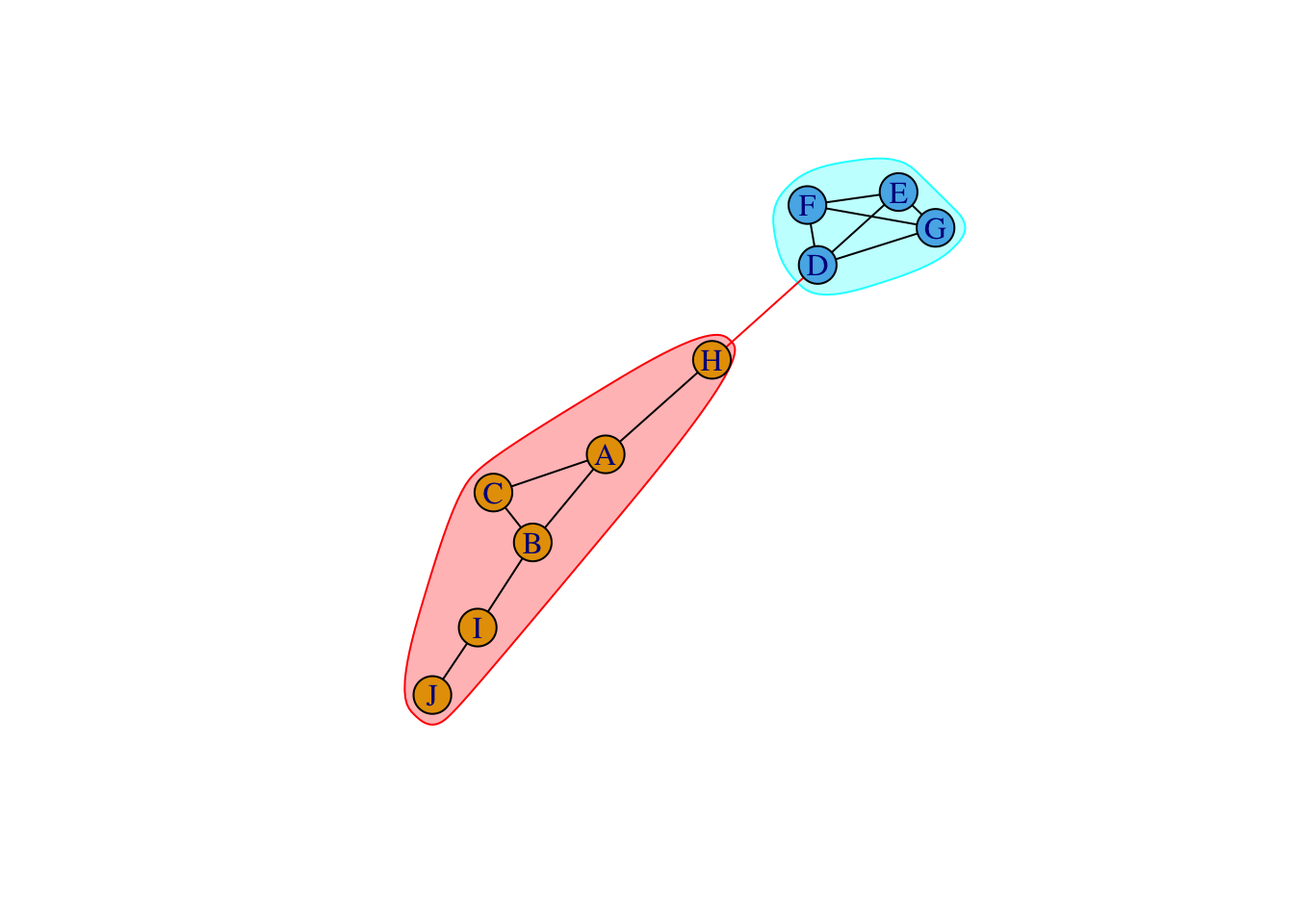3312 字

# 疫苗，节点免疫与出圈

``library(igraph)``
``````##
## Attaching package: 'igraph'``````
``````## The following objects are masked from 'package:stats':
##
##     decompose, spectrum``````
``````## The following object is masked from 'package:base':
##
##     union``````
``````g <- make_graph(~A:B:C-A:B:C, D:E:F:G-D:E:F:G, A-H-D, B-I,I-J)
set.seed(1)
plot(g, layout=layout_with_fr)````````````cluster <- edge.betweenness.community(g)
plot(cluster, g, layout=layout_with_fr)``````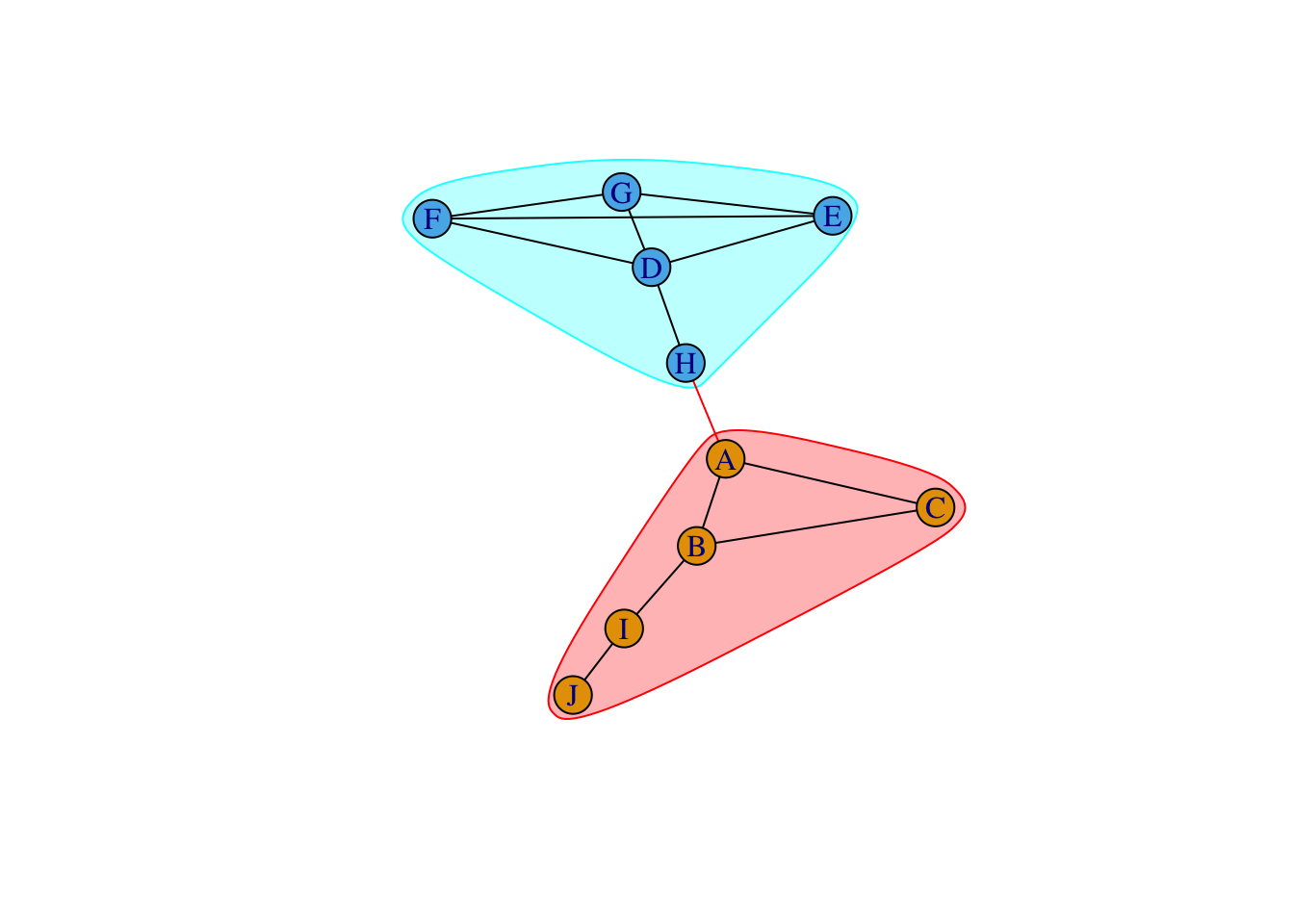``````# Clauset et al. 2004
cluster <- fastgreedy.community(g)
plot(cluster, g, layout=layout_with_fr)``````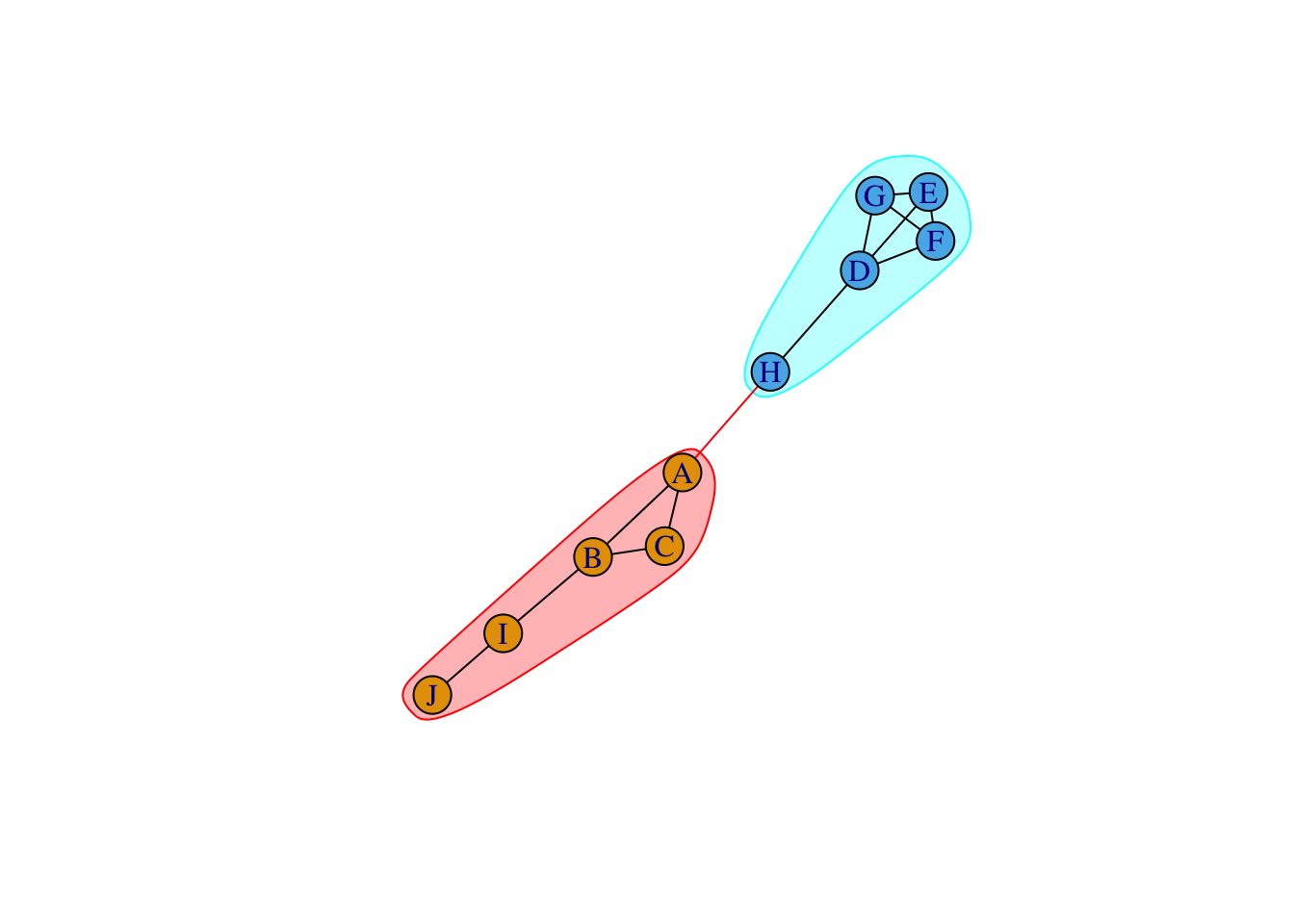``````# Pons & Latapy 2005
cluster <- walktrap.community(g)
plot(cluster, g, layout=layout_with_fr)````````````# Reichardt & Bornholdt 2006
cluster <- spinglass.community(g)
plot(cluster, g, layout=layout_with_fr)````````````# Newman 2006
plot(cluster, g, layout=layout_with_fr)````````````# Raghavan et al. 2007
cluster <- label.propagation.community(g)
plot(cluster, g, layout=layout_with_fr)``````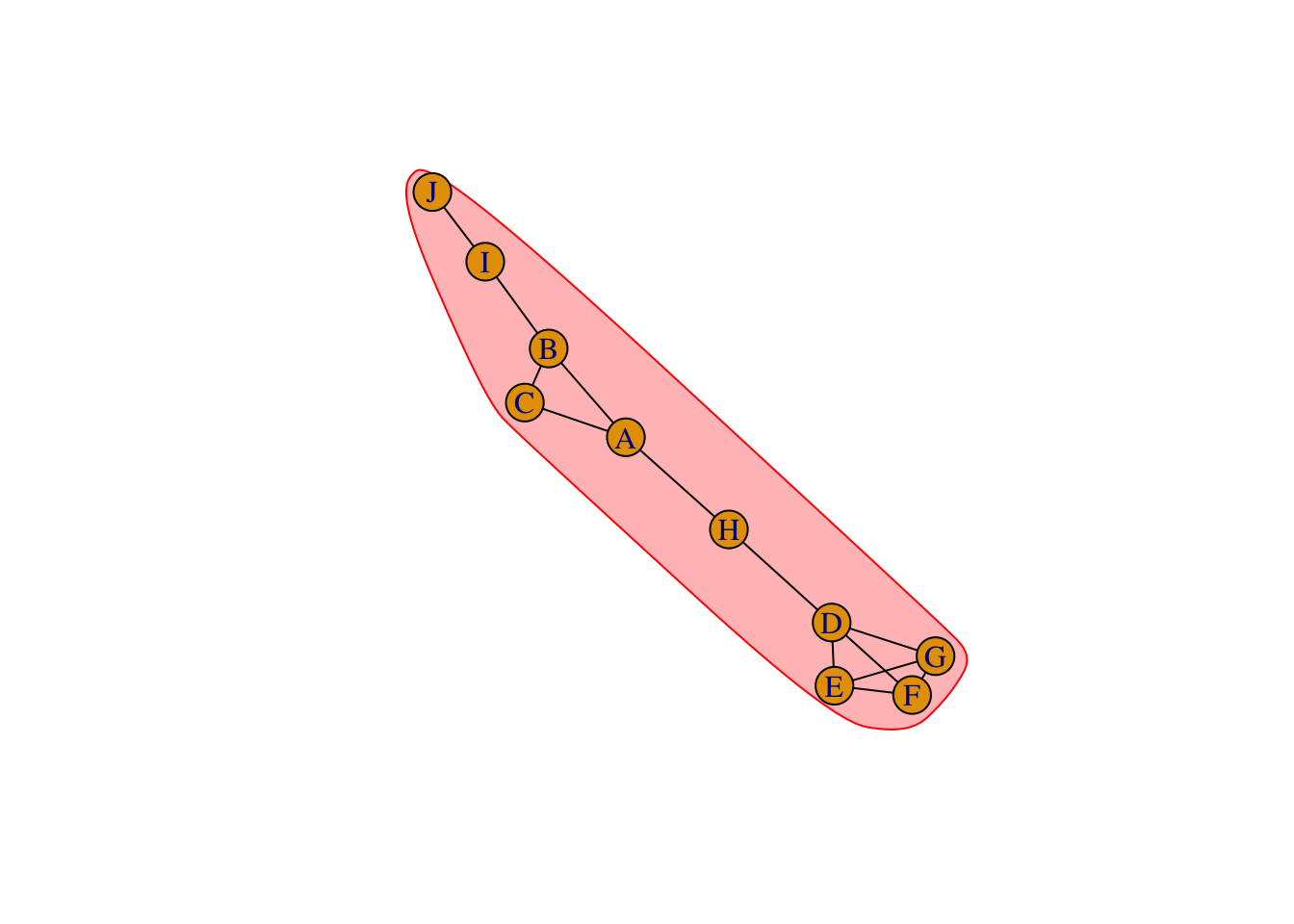``````# Blondel et al. 2008
cluster <- cluster_louvain(g)
plot(cluster, g, layout=layout_with_fr)``````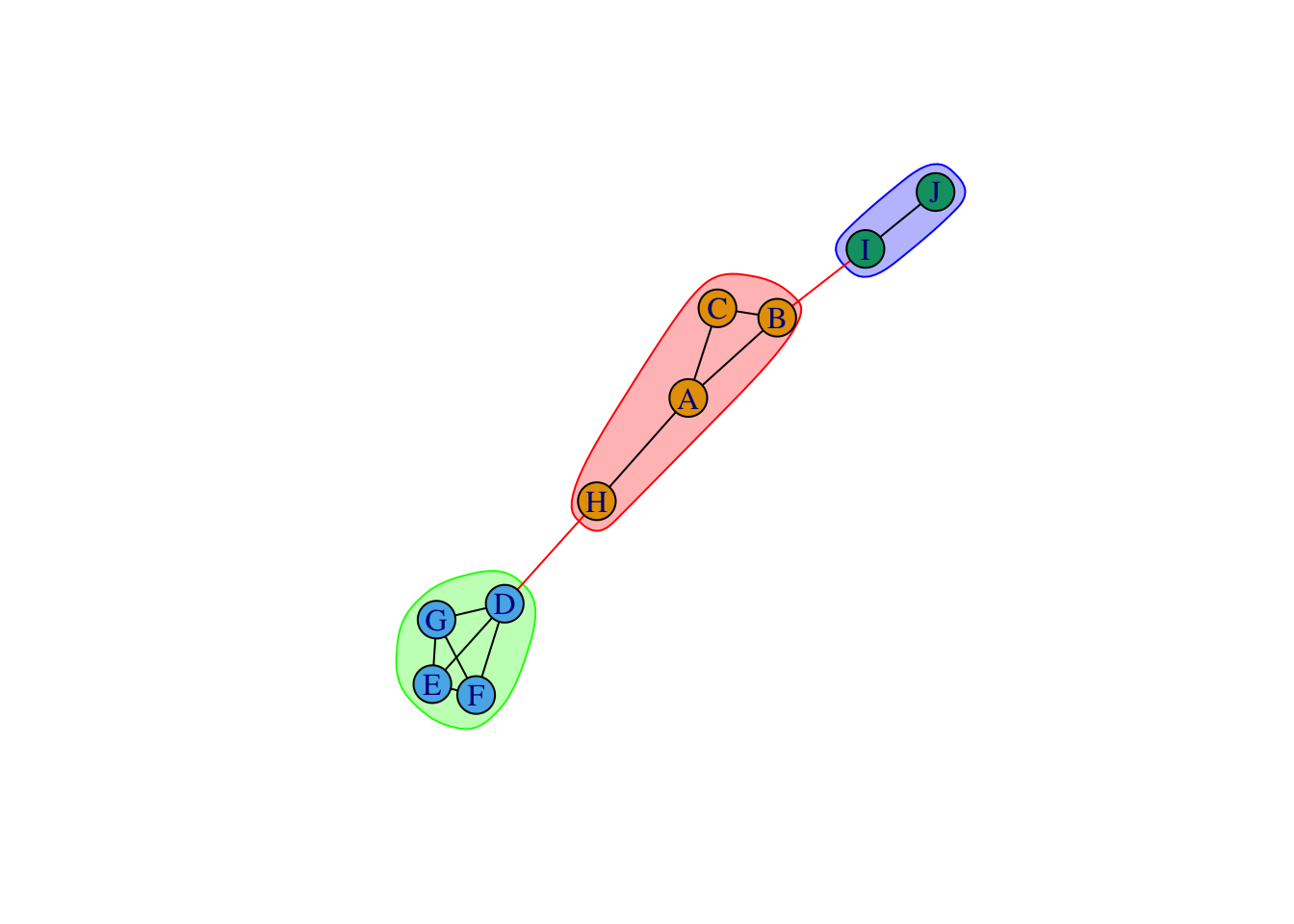``````# Rosvall et al. 2009
cluster <- cluster_infomap(g)
plot(cluster, g, layout=layout_with_fr)``````